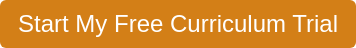Curriculum Areas
State Resources

# Medical Mathematics

This module provides a review of math skills frequently used by health care workers.Students review basic numerical concepts, including whole numbers, decimals, fractions, percentages, estimates, and measurements using the household, metric, and apothecary systems. Students practice using ratios and proportions to convert between measuring systems and calculate medication dosages, read labels and prescriptions, and learn how temperature and time are used in health care. Students record an Intake and Output chart and a TPR graph.

Unit: Basic Math Review Worksheet

Description
In this activity, students use a worksheet to review basic mathematical functions that are needed for this unit. This activity can be done individually, in teams or as a class.

Unit: Number Basics

Description
In this unit, students will learn about: how mathematics is used in the medical profession as they review Roman numerals, whole numbers, and number parts.

Lessons
1. Medical Mathematics
2. Number Basics
3. Quiz

Unit: Key Calculations

Description
In this unit, students will learn about: key calculations using ratios, proportions, estimations, and rounding numbers as they reduce ratios to lowest terms, use proportions to solve for unknowns, round and average numbers, and see how estimating is useful to the health care worker.

Lessons
1. Ratios and Proportions
2. Estimating
3. Quiz

Unit: Measurement Systems

Description
In this unit, students will learn about: measurements using the English, metric system, and apothecary systems as they practice converting measurement units within each system and learn how to make conversions among these measuring systems.

Lessons
1. English System
2. Metric System
3. Apothecary System
4. Conversions
5. Quiz

Unit: Medications

Description
In this unit, students will learn about: medications as they learn how to interpret labels on prescription drugs and calculate medication dosages and IV drip rates.

Lessons
1. Labels and Prescriptions
2. Dosages
3. Parenteral Medication
4. Quiz

Unit: Temperature and Time

Description
In this unit, students will learn about: measurements for temperature and time as they convert between Fahrenheit and Celsius and measure, calculate, and record time.

Lessons
1. Temperature
2. Time
3. Quiz

Unit: Charts and Graphs

Description
In this unit, students will learn about: recording patient status by measuring and recording patient intake and output and adding information to a TPR graph.

Lessons
1. Intake and Output Chart
2. TPR Graph
3. Quiz

Unit: Reflection Questions and Discussion

Description
In this activity, students will journal about: making measurements in traditional and metric units; converting units between systems of measurements; and using fractions, decimals, and percentages. A class discussion can follow.

Unit: Review Worksheet

Description
In this activity, students review what they have learned by completing the medical math worksheet.

Unit: Current Event

Description
Students will review articles for information related to a topic in the module. Once an article is found, students complete a Current Event form.